# Research on Digital Image Intelligent Recognition Method for Industrial Internet of Things Production Data Acquisition

Research on Digital Image Intelligent Recognition Method for Industrial Internet of Things Production Data Acquisition
Shenzhen Polytechnic Mobile Internet Public Technology Service Platform, Shenzhen 518055, China

Shenzhen Decard Smartcard Tech Co., Ltd., Shenzhen 518055, China

Corresponding Author Email:
lcq@decard.com
Page:
2133-2139
|
DOI:
https://doi.org/10.18280/ts.390626
2 October 2022
|
Accepted:
7 December 2022
|
Published:
31 December 2022
| Citation

OPEN ACCESS

Abstract:

The real scene data of production images and videos collected by the perception layer of the industrial Internet of Things, which are shot under the conditions of lack of illumination, underexposure and insufficient contrast, need to be fully and efficiently utilized to ensure the smooth progress of the follow-up supervision, monitoring, detection and tracking of the industrial Internet of Things. Therefore, this paper studies the intelligent recognition method of digital images on production data collected by industrial Internet of Thing. Firstly, the video or image data collected by the industrial Internet of Things monitoring platform are preprocessed to achieve the purpose of image clarity and targeting. It includes constrained least square restoration and Lucy-Richardson restoration for image blur caused by defocus, and blind deconvolution restoration for image motion blur caused by vibration. The adaptive histogram equalization algorithm is described in detail, and it can enhance the global contrast of digital images collected by industrial Internet of Things while retaining the details of the target area as much as possible. Based on U-net convolution network, the target recognition model of digital images collected by industrial Internet of Things is constructed, and spatial convolution pooling pyramid and improved convolution module Inception are introduced to optimize the model. Experimental results verify the effectiveness of the model.

Keywords:

industrial Internet of Things, production images, digital image, image target recognition

1. Introduction

Industrial Internet of Things is the application of Internet of Things in the industrial field, and plays an important role in energy, transportation, manufacturing and other application fields [1-10]. Industrial Internet of Things is the key foundation of industrial Internet, covering cloud computing, network, edge computing and terminals, and opening up key data streams in industrial Internet from bottom to top [11-14]. Industrial Internet of Things is divided from structure into perception layer, communication layer, platform layer and application layer. The perception layer is mainly composed of sensors, visual sensing and PLC, which collects data such as temperature, humidity, image, sound wave stream and video stream and transmits them to the network layer to assist the upper management system in recording, analyzing and making decisions [15-20]. Therefore, it is particularly important to study the efficient use of production data collected by the perception layer of industrial Internet of Things [21-22]. Especially for the real scene data of images and videos shot under the conditions of lack of illumination, underexposure and insufficient contrast, if they cannot be effectively processed, it will bring great difficulties to the follow-up supervision, monitoring, detection and tracking of the industrial Internet of Things.

Zhang  introduces the artificial intelligence technology and its new development trend. Combined with the specific images of public facilities, on the basis of traditional methods, it improves the application of different computer manual recognition methods in image recognition processing, and analyzes and compares the processing and recognition methods through corresponding simulation software. Di  studies and designs a digital image recognition algorithm based on pattern recognition. Feature vectors include four-dimensional feature vectors, eight-dimensional feature vectors and two-dimensional feature vectors based on principal component analysis. Classification methods include K-nearest neighbor method, minimum distance method and fixed increment method. By combining different feature vectors and different classification methods, different classification results are obtained. At the same time, the advantages, disadvantages and accuracy of the three methods are compared. The results show that K-nearest neighbor method has high accuracy, is insensitive to outliers and is easy to implement. Yang et al.  mainly studies the intelligent traceability of digital images based on improved fuzzy c-means clustering analysis. This method can improve the recognition of information authenticity of data images, and is helpful to the development of data image information detection and recognition system based on improved fuzzy c-means clustering analysis. He et al.  analyzes Canny algorithm, which uses Gaussian filter to smooth the image to eliminate the influence of noise on the detection results. Therefore, based on modular intelligent image recognition, four directions in 3 × 3 neighborhood are used to calculate gradient amplitude and direction. This method of calculating gradient amplitude and direction considers the diagonal direction, improves the accuracy of edge location and suppresses some noises. From the experimental results, it can be seen that Canny edge detection algorithm can detect the edge of the object perfectly.

A digital image intelligent recognition method with high robustness, high accuracy and perfect performance for production data collected by industrial Internet of Things is the key to obtain accurate results for various tasks such as follow-up supervision, detection and tracking of industrial Internet of Things, and is also an important guarantee to improve the supervision and operation efficiency of industrial Internet of Things monitoring platform. Therefore, this article has carried out relevant research. First of all, the second chapter of the article preprocesses the video or image data collected by the monitoring platform of the industrial Internet of Things in order to achieve the purpose of image clarity and targeting. It includes constrained least square restoration and Lucy-Richardson restoration for image blur caused by defocus, and blind deconvolution restoration for image motion blur caused by vibration. In the third chapter, the adaptive histogram equalization algorithm is described in detail, and can enhance the global contrast of digital images collected by industrial Internet of Things while retaining the details of the target area as much as possible. In the fourth chapter, based on U-net convolution network, the target recognition model of digital images collected by industrial Internet of Things is constructed, and spatial convolution pooling pyramid and improved convolution module Inception are introduced to optimize the model. Experimental results verify the effectiveness of the model.

2. Restoration Algorithm of Digital Image Collected by Industrial Internet of Things

## 1.pngFigure 1. Restoration flow chart of digital images collected by industrial Internet of Things

In order to improve the quality of digital images collected by the industrial Internet of Things and the recognizability of targets in the images, it is more conducive to the observation or further analysis and processing of the monitoring platform. Because ordinary monitoring images are usually acquired based on 30-frame, 1920*1080P camera equipment, its image quality is easily interfered by light changes, light and shade contrast, noise, equipment jitter, lens stains, etc. Therefore, in this chapter, the video or image data collected by the industrial Internet of Things monitoring platform is preprocessed to achieve the purpose of image clarity and targeting. Figure 1 shows the restoration flow chart of digital images collected by industrial Internet of Things.

Aiming at the image blurring caused by defocus, this article carries out constrained least square restoration and Lucy-Richardson restoration on the digital images collected by industrial Internet of Things. The precondition of image restoration using constrained least square method is that the image, blur type, noise type and its additive and multiplicative property are known. Assuming that the linear operator of f is represented by W and the optimal estimate of g is represented by g*, the function ||Wg*||2+x(||h-Fg*||2-||m||2) can be minimized, where g* can be expressed as g*=(FT FWeW) -1FTh, where the Lagrangian operator satisfies α=x-1. The restoration process is to repeat the iterative constant x until it satisfies ||h-Hg*||2=||m||2.

This method fully considers the stationary random process of image signal g and noise m, defines WTW =Sg-1Sm, and obtains g*=(FTF+αSg -1Sm)-1FTh. It is assumed that the noise and autocorrelation coefficients of the image are represented by Sg=T{ggT} and Sm=P{mmT}. The power spectra of image and noise are represented by Fourier Transforms Sg(a,b) and Sm(a,b)of Rg (v,v) and Rm(v,u), respectively. The signal-to-noise ratio is represented by NR=Rg(v,u)/Rm(v,u), and the parameter of adjustable Wiener filter is represented by B. The following formula characterizes the principle of Wiener filter restoration:

$\hat{G}(v, u)=\frac{1}{F(v, u)} \frac{|F(v, u)|^2}{|F(v, u)|^2+\alpha \frac{1}{N R}} H(v, u)+M(v, u)$    (1)

When Lagrange operator α is less than 1, it shows that the processing process is effective.

Lucy-Richardson algorithm based on planar Bayesian theory is an improved Wiener filter restoration algorithm, which can be implemented in MATLAB. For the restoration of digital images collected by industrial Internet of Things, it is assumed that the image estimates generated during the f+1 iteration and the f iteration are represented by gm+1(a,b) and gm(a,b), respectively. When the algorithm obeys Poisson distribution, it has the following iterative equation:

$g(a, b)^{l+1}=g(a, b)^l\left[\left[\frac{h(a, b)}{f(a, b) \otimes g(a, b)^k} \oplus f(a, b)\right.\right.$   (2)

Lucy-Richardson algorithm makes gm(a,b) converge to g(a,b) by iterative process of image itself.

In case of the motion blur of the digital images collected by the industrial Internet of Things caused by vibration, the direction and amplitude of vibration are uncertain, so it is inappropriate to use the previous algorithm to restore the images under the working conditions with high time requirements. In this article, the blind deconvolution restoration method, which can estimate the point spread function, is used to restore the collected digital images. Figure 2 shows the execution flow of blind deconvolution restoration method. Assuming that the digital images collected by the original industrial Internet of Things, the point spread function and the power spectrum of noise are represented by Rgg(v,v), Rff(v,u) and Rmm(v,u), respectively, the following formula gives the calculation formula of the algorithm:

$\hat{G}(v, u)=\frac{1}{F(v, u)} \frac{\|F(v, u)\|^2 H(v, u)}{\|F(v, u)\|^2+R_{m m}(v, u) / R_{g g}(v, u)}$   (3)

$F(v, u)=\frac{1}{\hat{G}(v, u)} \frac{\|\hat{G}(v, u)\|^2 H(v, u)}{\|\hat{G}(v, u)\|^2+R_{m m}(v, u) / R_{g g}(v, u)}$   (4)

## 2.png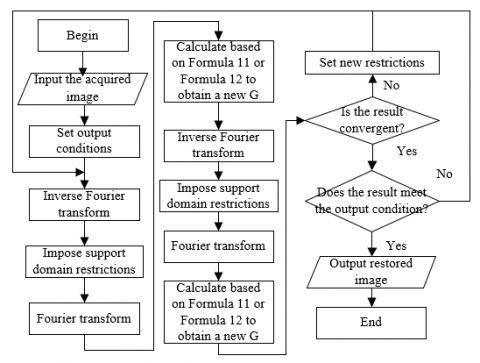Figure 2. Execution flow of blind deconvolution restoration method

3. Enhancement Method of Digital Images Collected by Industrial Internet of Things

In view of the infrared images that often appear in digital images collected by industrial Internet of Things, in order to make up for the loss of image gray level in the shooting process, the insufficient frequency of spatial sampling and the low resolution of the image caused by optical diffraction, this article selects adaptive histogram equalization algorithm to enhance the global contrast of digital images collected by industrial Internet of Things while retaining the details of the target area as much as possible.

Let the probability distribution function after Gaussian filtering be represented by o'(GUl), the filtering window size by 2q1+1, and the one-dimensional Gaussian kernel function by L(j), then:

$l(a)=\frac{1}{\sqrt{2 \pi} \rho_1} t^{-\frac{\left(a-a_0\right)^2}{2 \rho_1}}$  (5)

$o^{\prime}\left(G U_l\right)=\sum_{j=l-q_1}^{l+q_1} o\left(G U_j\right) \cdot l(j), l=q_1, q_1+1, \ldots, K-q_1-1$    (6)

Then, the local histogram of digital image is roughly smoothed by the data smoothing method based on LOWESS, and the minimum value of the new image probability density function is obtained based on the following formula:

$o\left(G U_l\right)=$$\left\{\begin{array}{l}\min \text { imum, } o *\left(G U_l\right)= \min \left(o *\left(G U_{l-0.5\left(q_2-1\right)}\right), \ldots, o *\left(G U_{l-0.5\left(q_2+1\right)}\right)\right) \\ \text { non- } \min \text { mum,others }\end{array}\right.$  (7)

In order to distinguish the foreground and background areas of digital images collected by the industrial Internet of Things, let the total number of gray levels and the cumulative density function in the interval [ni,ni+1] be represented by Mi and Di respectively, the gray density of each interval can be calculated based on the following formula:

$\Pi_i=\frac{D_i}{M_i}, i=1,2,3, \ldots, n-1$  (8)

If the value of Πi is small, it can be judged as the foreground area, and if it is large, it can be judged as the background area. Based on the theory of local maximum intra-class variance, a adaptive threshold Π* can be obtained to judge the foreground and background areas, and the histograms of the two types of areas can be distinguished by the following formula:

$\left\{\begin{array}{l}\Pi_i \leq \Pi^*,\left[n_i, n_{i+1}\right] \in \text { background area } \\ \Pi_i>\Pi^*,\left[n_i, n_{i+1}\right] \in \text { background area }\end{array}\right.$   (9)

Further, sub-histograms of foreground and background areas can be processed based on different enhancement strategies.

4. Intelligent Recognition of Digital Image Targets Collected by Industrial Internet of Things

Compared with traditional digital image processing methods, it is faster and more accurate to use deep learning to intelligently identify targets from digital images collected by industrial Internet of Things. Based on U-net convolution network, this chapter constructs the target recognition model of digital images collected by industrial Internet of Things. This model can realize the automatic segmentation of digital images collected by the industrial Internet of Things, and lay a foundation for the follow-up supervision, monitoring, detection and tracking of the industrial Internet of Things.

Whether the mean square error loss function or the average absolute error loss function is used, the error function of the model needs to conform to the hypothesis of Gaussian distribution or Laplace distribution; otherwise it will lead to poor error loss effect. This article uses GDL (Generalized Dice Loss) loss function, which is commonly used in medical image segmentation, to obtain strong small target recognition ability of digital images collected by industrial Internet of Things. Assuming that the actual value of the pixel of category k at the m-th position of the digital image collected by the industrial Internet of Things is represented by skm, the corresponding prediction probability value is represented by okm, and the weight of each category is represented by qk, the following formula gives the functional expression:

$G D_{-} L o s s=1-2 \frac{\sum_{k=1}^2 \quad q_k \sum_m s_{k m} o_{k m}} \quad{\sum_{k=1}^2 \quad q_k \sum_m s_{k m} \quad +o_{k n}}$    (10)

qk can be obtained based on the following formula:

$q_k=\frac{1}{\sum_{i=1}^m s_{k m}{ }^2}$   (11)

For the target to be recognized in the digital image collected by the industrial Internet of Things, each classified sample has a GDL loss function, and qk is inversely proportional to the image area size. To a certain extent, this reduces the influence of the area occupied by the target in the digital images collected by the industrial Internet of Things on the recognition effect of the recognition model, and is suitable for product quality supervision or the recognition and classification of small defects of the target. In order to fully consider the characteristics of all classified samples, this article uses the full gradient descent algorithm to optimize the recognition model. Let the number of samples be represented by n, and the following formula gives the expression of the target function:

$M B\left(\omega_0, \omega_1\right)=\frac{1}{2 n} \sum_{i=1}^n\left(f_\omega\left(a^{(i)}\right)-b^{(i)}\right)^2$   (12)

The two-sided derivation of the above formula is as follows:

$\frac{\partial M B\left(\omega_0, \omega_1\right)}{\partial \omega_j}=\frac{1}{n} \sum_{i=1}^n\left(f_\omega\left(a^{(i)}\right)-b^{(i)}\right) a_j^{(i)}$   (13)

In the process of model iteration, the weights are continuously updated, then:

$\omega_j^{\prime}=\omega_j-\mu \frac{1}{n} \sum_{i=1}^n\left(f_\omega\left(a^{(i)}\right)-b^{(i)}\right) a_j^{(i)}$   (14)

U-net convolution network consists of convolution layer, pooling layer and full connection layer. In order to accurately extract the target features of digital images collected by the industrial Internet of Things, multi-layer convolution layers are usually set in the network model, and multiple convolution kernels in the convolution layer are used for convolution operation to obtain the target feature response map. Set the integrable continuous functions on ℝ as g(a) and h(a), the following formula gives the convolution calculation formula:

$\operatorname{conv}(e)=\int_{-\infty}^{\infty} g(a) h(a-e) d a$   (15)

In order to improve the performance of target recognition model for digital images collected by industrial Internet of Things, this article introduces spatial convolution pooling pyramid and improved convolution module Inception to optimize the structure of U-net convolution network.

Pyramid pooling of porous space consists of two parts, namely, cavity convolution module and pyramid pooling module. Figure 3 shows the structure diagram of pyramid pooling of porous space. This module is designed to adjust the receptive field of convolution kernel without changing parameters and image resolution.

## 3.png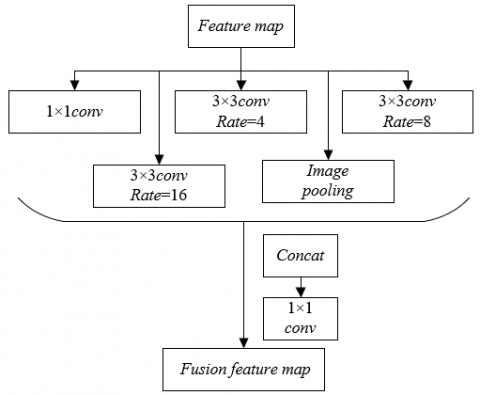Figure 3. Structure diagram of pyramid pooling of porous space

The Inception module is designed to realize feature extraction of digital images collected by industrial Internet of Things with different sizes through multiple convolution kernels. Figure 4 shows the structure diagram of the improved convolution module Inception. Figure 5 shows the schematic diagram of the improved model architecture.

## 4.png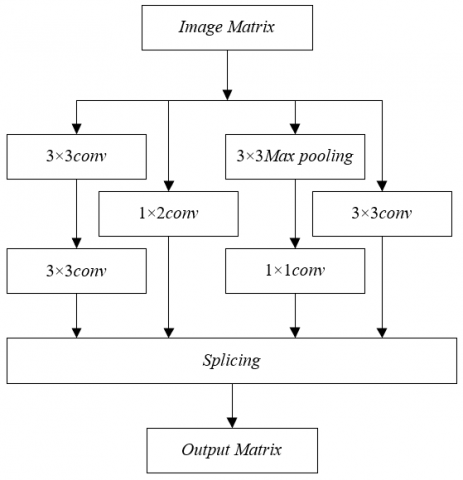Figure 4. Structure diagram of improved convolution module Inception

## 5.jpgFigure 5. Schematic diagram of improved model architecture

In view of the difficulty in obtaining digital images collected by the public industrial Internet of Things, this article expands based on the existing 400 digital images of industrial production process. The horizontal image expansion formula and the vertical image expansion formula are given by the following formula:

$N E_{-} I M(a, b)=O L_{-} I M(W I-a-1, b)$   (16)

$N E_{-} I M(a, b)=O L_{-} I M(a, H E-b-1)$   (17)

5. Experimental Results and Analysis

## 6.png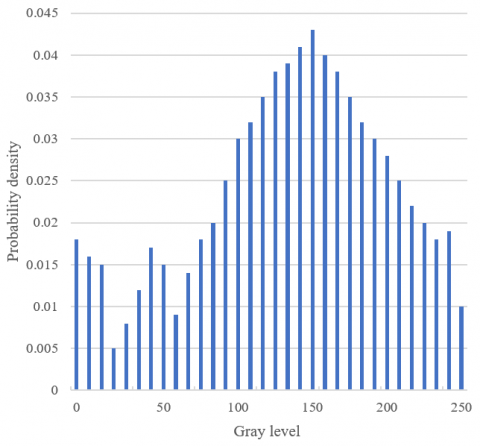Figure 6. Digital image histogram after adaptive histogram equalization

Figure 6 shows the digital image histogram after adaptive histogram equalization. As can be seen from the figure, after adaptive histogram equalization, the gray level range of digital images collected by industrial Internet of Things is expanded to [0, 255], the contrast and brightness of the image are enhanced, and the details of the target features in the image can be clearly seen, but at the same time, the image noise is also amplified, so it is necessary to restore the image before histogram equalization. Figure 7 shows the fitting curve of probability density function after image restoration processing.

## 7.png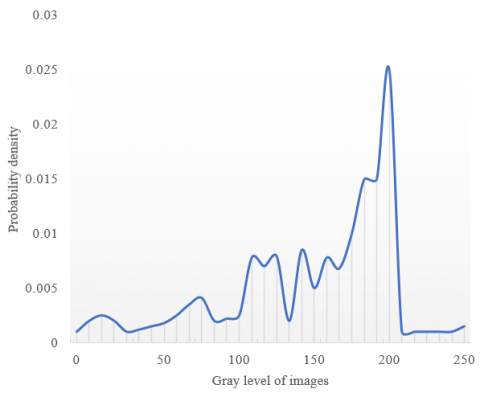Figure 7. Probability density function after image restoration processing

The quality of restored and enhanced images is quantitatively analyzed, and the evaluation indexes selected include MSE, PSNR and MSSIM. The restored and enhanced digital images are compared with their corresponding high-quality gray-scale digital images, and three image preprocessing methods are set: only enhancement without restoration, only restoration without enhancement, and enhancement and restoration without smoothing. The experimental results are given in Table 1.

Table 1. Image quality evaluation results of restoration and enhancement algorithms

 Target area number Method I Method II Method III Method used MSE 1 6.528 5.314 8.362 3.625 2 14.205 8.025 15.927 7.392 3 8.269 6.274 11.041 4.015 PSNR 1 11.025 13.602 8.062 13.527 2 7.419 8.274 6.341 9.514 3 9.528 14.629 8.295 13.627 MSSIM 1 0.436 0.314 0.539 0.439 2 0.517 0.459 0.547 0.527 3 0.609 0.431 0.619 0.641

It can be seen from the above table that the images processed by the restoration and enhancement algorithm have obtained smaller MSE values and larger PSNR values and MSSIM values, which verifies that the digital images collected by the industrial Internet of Things after restoration and enhancement are closest to the real images of industrial production scenes, and is beneficial to further intelligent image recognition.

## 8.png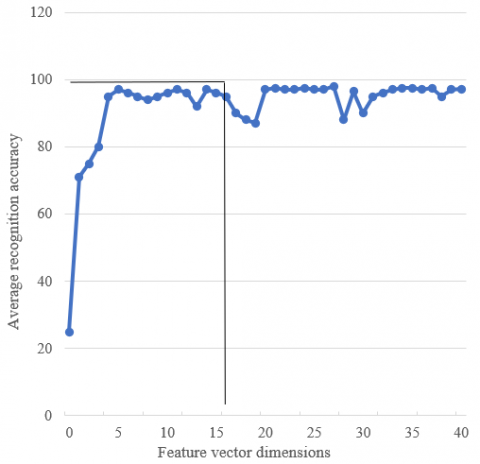Figure 8. Accuracy of target recognition under different feature vector dimensions

After the feature vector expression of image targets is determined, in order to fully consider the features of all classified samples, it is necessary to select the length of feature vector according to the actual situation. In order to find the best feature vector dimension, this article designs related experiments to test the target recognition accuracy under different feature vector dimensions. The corresponding experimental results are given in Figure 8.

It can be seen from the figure that with the increase of feature vector dimension, the average recognition accuracy of image targets gradually increases and tends to be stable. After analyzing the variation curve of the average recognition accuracy, this article decides to take the feature vector dimension as 15, under which the recognition accuracy is ideal and the computational complexity of the model is moderate.

Table 2. Performance comparison of different target recognition models

 Index Sample No. Traditional U-net Faster R-CNN Model used Accuracy 1 0.652 0.569 0.857 2 0.614 0.427 0.841 3 0.736 0.536 0.836 4 0.741 0.574 0.859 5 0.759 0.596 0.827 6 0.725 0.538 0.802 Recall rate 1 0.847 0.836 0.901 2 0.826 0.814 0.947 3 0.814 0.825 0.958 4 0.803 0.858 0.936 5 0.827 0.836 0.901 6 0.825 0.827 0.914 Cross-merger ratio 1 0.725 0.734 0.857 2 0.869 0.749 0.841 3 0.825 0.735 0.825 4 0.835 0.758 0.804 5 0.847 0.736 0.869 6 0.725 0.795 0.837

In order to further verify the effectiveness of the target recognition model of digital images collected by the industrial Internet of Things built in constructed herein, this article compares the performance of the improved U-net model, the traditional Faster R-CNN model and this model. The comparison results are given in Table 2. It can be seen from the table that the maximum target recognition accuracy of this model can reach 0.958, while the traditional U-net model and Faster R-CNN model are only 0.847 and 0.858. At the same time, the recall rate of this model is higher and the false detection rate is lower than the other two models. Compared with the traditional U-net model before the improvement, this model introduces spatial convolution pooling pyramid and improved convolution module Inception, which expands the receptive field of convolution layer, ensures that the segmentation result of the images is closer to the real scene of industrial production, and has stronger recognition ability to the target area.

6. Conclusion

This article studies the intelligent recognition method of digital images on production data collected by industrial Internet of Things. Firstly, the video or image data collected by the industrial Internet of Things monitoring platform are preprocessed to achieve the purpose of image clarity and targeting. It includes constrained least square restoration and Lucy-Richardson restoration for image blur caused by defocus, and blind deconvolution restoration for image motion blur caused by vibration. The adaptive histogram equalization algorithm is described in detail, and it can enhance the global contrast of digital images collected by industrial Internet of Things while retaining the details of the target area as much as possible. Based on U-net convolution network, the target recognition model of digital images collected by industrial Internet of Things is constructed, and spatial convolution pooling pyramid and improved convolution module Inception are introduced to optimize the model. Experimental results verify the effectiveness of the model.

The experimental results show the digital image histogram after adaptive histogram equalization and the fitting curve of probability density function after image restoration. The quality of restored and enhanced images is quantitatively analyzed, and the effectiveness of the proposed restoration and enhancement algorithms is verified. Comparing the performance of different target recognition models further verifies the effectiveness of the target recognition model of digital images collected by the industrial Internet of Things built in constructed herein.

Acknowledgments

This work was supported in part by the Shenzhen key technology research project: key technology research and development of high-speed and high-precision vertical five-axis machining center (Grant No.: 2019N048) and in part by the Project of Science and Technology of Shenzhen (Grant No.: JSGG20180504165556479).

References

 Subramani, C., Usha, S., Patil, V., Mohanty, D., Gupta, P., Srivastava, A.K., Dashetwar, Y. (2020). IoT-based smart irrigation system. Cognitive Informatics and Soft Computing, 1040: 357-363. https://doi.org/10.1007/978-981-15-1451-7_39

 Robles, M.I., Narendra, N.C., Kiviranta, S.M. (2020). Pragmatic distance in IoT devices. IEEE Transactions on Network and Service Management, 17(4): 2731-2743. https://doi.org/10.1109/TNSM.2020.3008840

 Mufti, R., Khatri, K., Bhardwaj, S., Gupta, P. (2020). Design of IoT-based SmartMat. Smart Systems and IoT: Innovations in Computing, 141: 39-49. https://doi.org/10.1007/978-981-13-8406-6_5

 Sisinni, E., Ferrari, P., Carvalho, D.F., Rinaldi, S., Marco, P., Flammini, A., Depari, A. (2019). LoRaWAN range extender for Industrial IoT. IEEE Transactions on Industrial Informatics, 16(8): 5607-5616. https://doi.org/10.1109/TII.2019.2958620

 Beytur, H.B., Baghaee, S., Uysal, E. (2020). Towards AoI-aware smart IoT systems. In 2020 International Conference on Computing, Networking and Communications (ICNC), 353-357. https://doi.org/10.1109/ICNC47757.2020.9049792

 Panda, S.S., Mohanta, B.K., Dey, M.R., Satapathy, U., Jena, D. (2020). Distributed ledger technology for securing IoT. In 2020 11th International Conference on Computing, Communication and Networking Technologies (ICCCNT), 1-6. https://doi.org/10.1109/ICCCNT49239.2020.9225333

 Gelenbe, E., Fröhlich, P., Nowak, M., Papadopoulos, S., Protogerou, A., Drosou, A., Tzovaras, D. (2020). IoT network attack detection and mitigation. In 2020 9th Mediterranean Conference on Embedded Computing (MECO), 1-6. https://doi.org/10.1109/MECO49872.2020.9134241

 Jang, H., Choi, S., Kwon, E., Kwon, C. (2020). IoT device auto-tagging using transformers. In 2020 12th International Conference on Advanced Infocomm Technology (ICAIT), 47-50. https://doi.org/10.1109/DCOSS49796.2020.00078

 Heeger, D., Plusquellic, J. (2020). Analysis of IoT authentication over LoRa. In 2020 16th International Conference on Distributed Computing in Sensor Systems (DCOSS), 458-465.

 Prasad, B. (2020). Product development process for IoT-ready products. Concurrent Engineering, 28(2): 87-88. https://doi.org/10.1177/1063293X20932618

 Li, Y., Liang, W., Xu, W., Jia, X. (2020). Data collection of IoT devices using an energy-constrained UAV. In 2020 IEEE International Parallel and Distributed Processing Symposium (IPDPS), 644-653. https://doi.org/10.1109/IPDPS47924.2020.00072

 Alaghehband, A., Ziyainezhad, M., Sobouti, M.J., Seno, S.A.H., Mohajerzadeh, A.H. (2020). Efficient fuzzy based UAV positioning in IOT environment data collection. In 2020 10th International Conference on Computer and Knowledge Engineering (ICCKE), 585-591. https://doi.org/10.1109/ICCKE50421.2020.9303618

 Tao, M., Li, X., Yuan, H., Wei, W. (2020). UAV-Aided trustworthy data collection in federated-WSN-enabled IoT applications. Information Sciences, 532: 155-169. https://doi.org/10.1016/j.ins.2020.03.053

 Kim, T., Qiao, D. (2020). Energy-efficient data collection for IoT networks via cooperative multi-hop UAV networks. IEEE Transactions on Vehicular Technology, 69(11): 13796-13811. https://doi.org/10.1109/TVT.2020.3027920

 Ma, J., Shi, S., Gu, S., Zhang, N., Gu, X. (2020). Age-optimal mobile elements scheduling for recharging and data collection in green IoT. IEEE Access, 8: 81765-81775. https://doi.org/10.1109/ACCESS.2020.2990931

 Aljohani, T., Zhang, N. (2020). A secure and privacy-preserving data collection (SPDC) framework for IoT applications. In International Conference on Critical Information Infrastructures Security, 12332: 83-97. https://doi.org/10.1007/978-3-030-58295-1_7

 Zhang, L., Li, F., Wang, P., Su, R., Chi, Z. (2021). A blockchain-assisted massive IoT data collection intelligent framework. IEEE Internet of Things Journal. 9(16): 14708-14722. https://doi.org/10.1109/JIOT.2021.3049674

 Khodaparast, S.S., Lu, X., Wang, P., Nguyen, U.T. (2022). Deep reinforcement learning based data collection in IoT networks. In 2022 IEEE Wireless Communications and Networking Conference (WCNC) 818-823. https://doi.org/10.1109/WCNC51071.2022.9771616

 Liu, Y., Chin, K.W., Yang, C. (2021). Link Scheduling for data collection in Multihop backscatter IoT wireless networks. IEEE Internet of Things Journal, 9(3): 2215-2226. https://doi.org/10.1109/JIOT.2021.3091144

 Goulart, A., Pinto, A.S.R., Boava, A., Branco, K. (2022). Data collection in an IoT Off-Grid environment systematic mapping of literature. Sensors, 22(14): 5374. https://doi.org/10.3390/s22145374

 Al-Khafaji, H.M.R. (2022). Data collection in IoT using UAV based on multi-objective spotted hyena optimizer. Sensors, 22(22): 8896. https://doi.org/10.3390/s22228896

 Wu, W., Sun, S., Shan, F., Yang, M., Luo, J. (2022). Energy-constrained UAV flight scheduling for IoT data collection with 60 GHz communication. IEEE Transactions on Vehicular Technology, 71(10): 10991-11005. https://doi.org/10.1109/TVT.2022.3184869

 Zhang, X. (2022). Application of artificial intelligence recognition technology in digital image processing. Wireless Communications and Mobile Computing, 2022: Article ID 7442639 | https://doi.org/10.1155/2022/7442639

 Di, S. (2021). Research on digital image recognition algorithm based on pattern recognition. In 2021 International Symposium on Computer Technology and Information Science (ISCTIS), 386-391. https://doi.org/10.1109/ISCTIS51085.2021.00085

 Yang, Y., Wang, Z., Liu, K., Zhu, H. (2021). Digital media image recognition method based on improved fuzzy c-means clustering analysis. In Journal of Physics: Conference Series, 1982(1): 012097. https://doi.org/10.1088/1742-6596/1982/1/012097

 He, X., Shao, J., Zhu, J. (2021). Research on digital image recognition algorithm based on modular intelligent image recognition. In 2021 IEEE International Conference on Advances in Electrical Engineering and Computer Applications (AEECA), 419-423. https://doi.org/10.1109/AEECA52519.2021.9574139